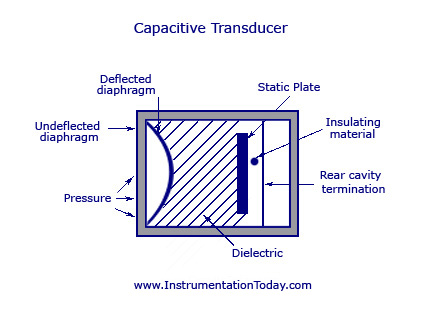UXDE dot Net Wordpress Themes

# Capacitive Transducers

To learn about a capacitive transducer, it is important to know the basics of a parallel plate capacitor. Being the simplest form of a capacitor, it has two parallel conducting plates that are separated to each other by a dielectric or insulator with a permittivity of Ε (for air). Other than paper, vacuum, and semi-conductor depletion region, the most commonly used dielectric is air.

Due to a potential difference across the conductors, an electric field develops across the insulator. This causes the positive charges to accumulate on one plate and the negative charges to accumulate on the other. The capacitor value is usually denoted by its capacitance, which is measured in Farads. It can be defined as the ratio of the electric charge on each conductor to the voltage difference between them.

The capacitance is denoted by C. In a parallel plate capacitor, C = [A*Er*9.85*1012 F/M]/d

A – Area of each plate (m)

d – Distance between both the plates (m)

Er – Relative Dielectric Constant

The value 9.85*1012 F/M is a constant denoted by Eo and is called the dielectric constant of free space.
From the equation it is clear that the value of capacitance C and the distance between the parallel plates,d are inversely proportional to each other. An increase of distance between the parallel plates will decrease the capacitance value correspondingly. The same theory is used in a capacitive transducer. This transducer is used to convert the value of displacement or change in pressure in terms of frequency.

You may also like: Capacitance Transducer

As shown in the figure below, a capacitive transducer has a static plate and a deflected flexible diaphragm with a dielectric in between. When a force is exerted to the outer side of the diaphragm the distance between the diaphragm and the static plate changes. This produces a capacitance which is measured using an alternating current bridge or a tank circuit.Capacitive Transducer

A tank circuit is more preferred because it produces a change in frequency according to the change in capacitance. This value of frequency will be corresponding to the displacement or force given to the input.

• It produces an accurate frequency response to both static and dynamic measurements.

• An increase or decrease in temperature to a high level will change the accuracy of the device.
• As the lead is lengthy it can cause errors or distortion in signals.1.nidhish shah

this is a very nice for our (basic) study…

thank you Mr. JOHN

……..er. nidhish shah(I.C.)

2.Mohit Ruhela

thankyou ,but it is not sufficient.

3.pikasha

how can we actually make a capacitve transducer?

4.Jairam singh Reddy

THIS IS ONLY INTRO ABOUT CAPACITIVE TRANSDUCER.

5.Jairam singh Reddy

this is insufficient.

6.arun kathariya

There is no any working principle given….

7.arun kathariya

I need the working principle of capacitive transducer….but it iz not given…..to much boring…???!

8.Levious

quite simple and easy to understand.thank you

9.liza

It is to good and vry importent of electronic engg.

10.hasrat roohani

thank you sir